In the verge of coronavirus pandemic, we are providing FREE access to our entire Online Curriculum to ensure Learning Doesn't STOP!

# Ex.3.1 Q1 Understanding Quadrilaterals Solution - NCERT Maths Class 8

Go back to  'Ex.3.1'

## Question

Given here are some figures.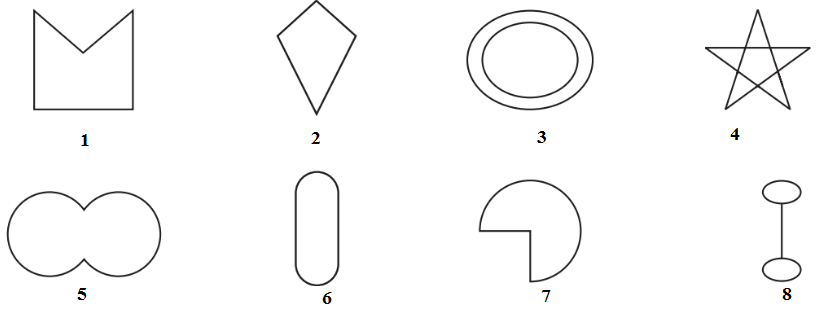Classify each of them on the basis of the following.

(a) Simple curve

(b) Simple closed curve

(c) Polygon

(d) Convex polygon

(e) Concave polygon

Video Solution
Ex 3.1 | Question 1

## Text Solution

Steps:

(a) Simple curve - A simple curve is a curve that does not cross itself.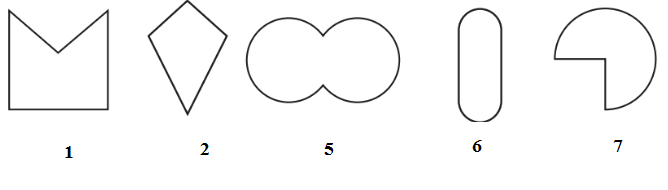(b) Simple closed curve -In simple closed curves the shapes are closed by line-segments or by a curved line.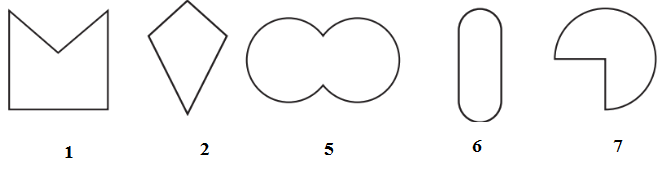(c) Polygon-A simple closed curve made up of only line segments is called a polygon.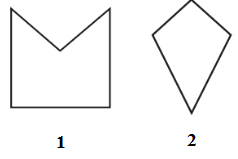(d) Convex polygon-A Convex polygon is defined as a polygon with no portions of their diagonals in their exteriors.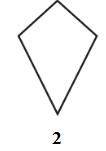(e) Concave polygon- A concave polygon is defined as a polygon with one or more interior angles greater than $$180^{\circ}.$$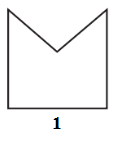Learn from the best math teachers and top your exams

• Live one on one classroom and doubt clearing
• Practice worksheets in and after class for conceptual clarity
• Personalized curriculum to keep up with school